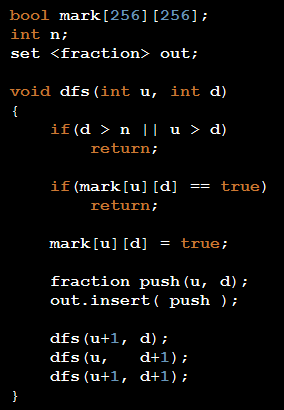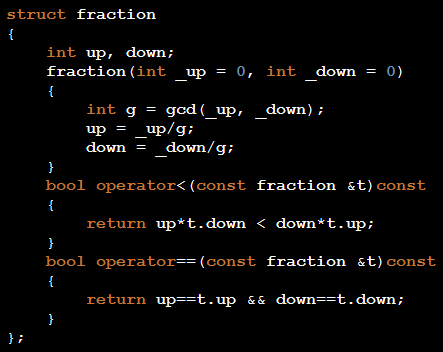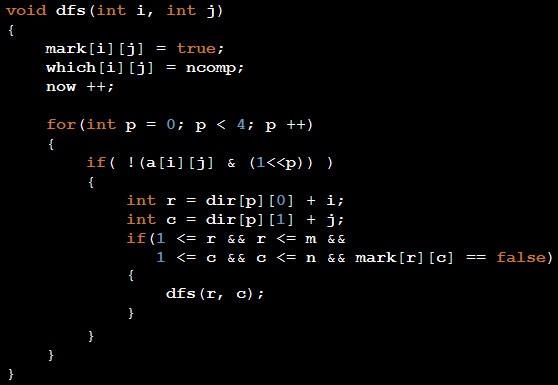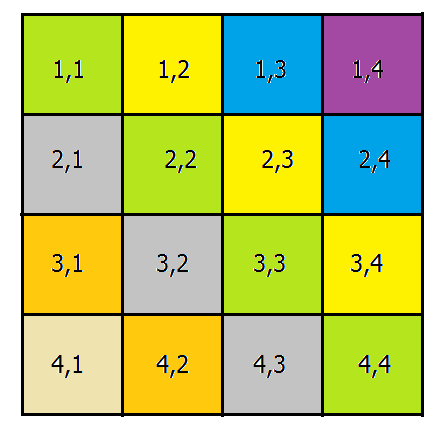## Sunday, December 1, 2013

### UVa - 11748 Rigging Elections

Link to the problem on UVa Online Judge

In this problem you must think more than you have to code, you must reason.
First of all if you think of candidates as nodes of a graph, then you'll understand that we are facing a tournament graph (A directed graph in which between any two vertices there is exactly one edge in one direction).
First we have to build the graph in O(n^2 * m), how do we build the graph? For any two candidates if we know voter j prefers which one in O(1) then we can build the graph in the desired order. After we read a voter's preference list we must save candidate i is the k-th person in this voter's list. Then for any two candidates and a voter we will understand the one who he prefers.
After building the graph we must think of different situations, situations that a candidate is winner. The simplest situation that may come to mind is a cycle. In a cycle every node can be a winner. If you look closer each node of a Strongly Connected Component can be a winner. What's the relation between two SCC's? If edges are from SCC A to SCC B, then each node of SCC A can be a winner if no other SCC has edges to SCC A. So we must put these SCC's in topological order and anyone in the first SCC can be a winner.
So the desired candidate can be the winner if he is in the first SCC in the topological order.

Another solution to this problem comes from a similar reasoning and that is : if you have a path to any other node then you can be the winner. Think of this situation and try to understand that if you have a path to a node then you can win any person on this node, especially the last node on this path.
How do you know if you have a path to all the other nodes? You simply use DFS(desiredCandidate), and if all the other nodes are marked as seen, then you have a path to all the other nodes.

## Wednesday, November 20, 2013

### TIMUS - 1120 Sum of sequential numbers

Link to the problem on Timus Online Judge

The problem statement is completely straight forward with no hidden tricks :). Yeah yeah I know that you know problem statement is straight forward :), so let's talk about solution.
What do I do in a problem like this? I pay attention to range of input. Let's rewrite what problem wants for output :
• N = A + (A + 1) + … + (A + P − 1)
• N = P*A + 0 + 1 + 2 + ... + (P-1)
• N = P*A + (P-1)*P/2
What does a good teacher do when you are solving a problem? He asks you meaningful questions to lead you to the solution. When a good teacher is not available (most of the times) try to do this favor for yourself, ask yourself meaningful questions about input, so you can understand new aspects of the problem you are solving.

Can we iterate over all possible values for P? or What could be maximum value for P according to range of input? Yes, what is the maximum value of P if N=10^9. If you look at the equations, P would be less than square root of 10^9, about 33,000, that's good, cause if you can iterate over all possible values for P, then A would be known in the equation.
What is value of A if we know N and P? A = ( 2*N - (P-1)*P ) / (2*P) such that : numerator must be positive and numerator must be divisible by denominator, then you can pick maximum value over 33,000 possible values for P.

### TIMUS - 1207 Median on the plane

Link to the problem on Timus Online Judge

This is a Computational Geometry problem. No 3 points lie on the same line and this is a great help about our approach to the problem. Since no three points lie on the same line, if you choose point A there is another point B which line AB divides all points equally. I choose the first point according to dictionary order, then if you can sort other points in increasing order of angle relating to the first point, then the other point would be obvious.

As you see in the figure I've chosen point 0 and want to sort other points in the given order(so the answer would be point 4 in this case), Surprisingly the problem reduces to a sorting problem and we know we can sort in O(n log n). We need to write a comparator for two points, I mean we need to identify between two points x and y which one comes earlier in the sorted list?

And how do we write the comparator? If the cross product of vector x-v and x-y is negative then x-v-y are clockwise, otherwise they are counter-clockwise.

At last we use function comp to sort other points.
sort(v.begin()+1, v.end(), comp);

## Tuesday, November 19, 2013

### TIMUS - 1036 Lucky Tickets

Link to the problem on Timus Online Judge

First of all there are two kinds of input which the answer is zero, first if sum % 2 == 1 or second if sum/2 > n*9, in first case we can not divide sum in two parts equally, in second case if we put 9 in all positions we can not make sum/2.

This is a Dynamic Programming problem, state of our dp is : dp[i][sum] which means we are on digit i and we want to make sum, how do we do that? We have to find sub-problems related to this state.
dp[i][sum] = SUM{ dp[i-1][sum-k] for k = 0...9, st sum-k >= 0 }
The base case is that we know dp = 1, which means there is one way to make sum of zero with zero digits, and that is nothing! :) ( like combination(0, n) = 1 )

And what is the answer after calculating dp[i][sum]?
The answer is dp[n][sum/2]*dp[n][sum/2] (multiplication principle).

## Sunday, November 17, 2013

### TIMUS - 1017 Staircases

Link to the problem on Timus Online Judge

If you look closely to the structure of different staircases for a specific n, you might understand the recursive structure of them, I mean some staircases have common prefix and from a column their difference starts.
If you are familiar with Dynamic Programming and think about state of the dynamic programming, sooner or later you will find a solution with time complexity O(n^3).
Let's define our state as this : dp[min][rem] which means in this column we are standing at we need to put at least min bricks and we have rem bricks remained to use, so what do we do about this column we are standing at?
We test all possible values for this column -> dp[min][rem] = SUM{ dp[k+1][rem-k], such that k = min...rem } which means for the next column use at least k+1 bricks and you have rem-k bricks remained.
What about the basic state? What is basic state, The state which we know the answer and now more recursion is needed. when rem is equal to 0 or rem is equal to min, there is no need to recur again, the answer to this state is equal to 1.

## Saturday, November 16, 2013

### TIMUS - 1114 Boxes

Link to the problem on Timus Online Judge

After I read the problem statement, I tried to think of a popular technique. First I noticed the number of red balls and blue balls. I tried to fix the number of red balls and blue balls chosen to put in the boxes with two for loops. Now I have n boxes and A1 red balls and B1 blue balls which are the same and must be put in the boxes. Another observation is that the arrangement of red balls and blue balls are not related to each other, so the overall answer is the multiplication of the answer for red balls and blue balls, which is calculated separately.
Let's denote the number of balls in the i-th box with Xi, then the answer for red balls is the number of answers to this equation X1 + X2 + ... + Xn = A1 : st. Xi >= 0. This is a classic problem in combinatorics and the number of solutions to this equation is equal to Combination(n-1, A1 + n-1), the same holds for B1, and the number of solutions for Blue balls is Combination(n-1, B1 + n-1), so for this fixed A1 and fixed B1 the answer is Combination(n-1, A1 + n-1) * Combination(n-1, B1 + n-1).

But there is another Cute combinatorial solution which needs no for loops over the number of red balls and blue balls. Let's add another box, if some balls are not put in the first n boxes, then we put it in the last box. So the answer for Red balls is the number of solutions to this equation : X1 + X2 + ... + Xn + X(n+1) = A : st Xi >= 0. Answer to this problem is Combination(n, A+n), and finally the over all answer is Combination(n, n+A) * Combination(n, n+B).

There is another solution to this problem using Dynamic Programming.
The state to our solution in DP is dp[boxi][a][b], we are on boxi, we have a red balls and b blue balls, we choose how many balls we want for boxi.
dp[boxi][a][b] = SUM{ dp[boxi+1][a-i][b-j]  : such that i=0...a  j=0...b }
and we know dp[n+1][xx][yy] = 1, it means we have filled the first n boxes and there are xx red balls and yy blue balls chosen to be out of boxes :)

## Wednesday, September 18, 2013

### POJ - Drainage Ditches

Link to the problem on Peking Online Judge

This is a primitive and classic Maximum Flow Problem.
We have a weighted directed graph in this problem, and we need to find maximum flow from node 1 to node M.

### POJ - Optimal Milking

Link to the Problem on Peking Online Judge

If you look closer to the problem statement and model it on a piece of paper, we have C cows each of which must be assigned to one of the K milking machines, so that the maximum distance walked by one of the cows is minimum. The bold phrase is one of the most important keys to problems which are solved using binary search + some other algorithm. Minimizing Maximum Distance.

In this problem we need to do a Binary Search over the maximum Distance and then check according to our limitation (at most M cows for each milking machine) whether we can find an assignment which obeys Maximum Distance which for now is fixed with Binary Search.

After these steps we know each cow can go to which machines. Here we have to find a good assignment of cows to milking machines if exists. We decide CowX goes to MilkingMachineY, but we are not sure that this decision is a good decision, so we make a plan to change our mind in the future if needed and send CowA to another milking machine (back edge in Max Flow). This behavior in the problem remembers us of Maximum Flow, which by each decision we are not completely sure of it's correctness and we make a plan to change it in the future if needed.
Edges of our graph :
• from source to each milking machine an edge with capacity M
• from each cow to sink and edge with capacity 1
• from each cow to each milking machine which mat[cowX][MMY] <= DIS an edge with capacity 1
Where does mat[i][j] come from? It is calculated using a floyd-warshall algorithm after reading input, mat[i][j] means the shortest path from entity i to entity j.
DIS comes from our Binary Search. After making the graph we run Max Flow on it, if the flow is equal to C DIS is good and we may find another DIS1 which is less than DIS, otherwise we need to find another DIS2 which is greater than DIS (What we do in Binary Search depending on the flow - if...else)

## Thursday, January 10, 2013

### USACO - Ordered Fractions

One way to solve this problem is to construct all possible fractions, reduce all of them as possible, sort them and print them.

Another way is to use DFS.Lets start with (0,1) and see what can we build? (Check it yourself)
and fraction is something like this :### USACO - The Castle

Think about problem, what does the problem wants? We have a grid and we want to find the number of connected components in this grid, the largest component before breaking a wall, and the largest component after breaking a wall, we also need the wall itself.

To find connected components one can easily use DFS.
int dir = {{0, -1}, {-1, 0}, {0, 1}, {1, 0}};
To deal with neighbors I have this array 'dir', a[i][j] is the value of cell (i, j), I want to know whether I can go to the other cells connected to (i, j) or not.After DFS, I iterate over cells as the problem statement wants and check it's neighbors, if its neighbor is in another component I check the size of the merged component to the maximum size found.

### USACO - Checker Challenge

If you are familiar with n-queen problem, when you are reading the problem statement you will remember that problem, Yes we are facing a n-queen problem, we want to count different ways to put n queens in a n*n board.

For those not familiar with n-queen problem

The eight queens puzzle is the problem of placing eight chess queens on an 8×8 chessboard so that no two queens attack each other. Thus, a solution requires that no two queens share the same row, column, or diagonal. The eight queens puzzle is an example of the more general n-queens problem of placing n queens on an n×n chessboard, where solutions exist for all natural numbers n with the exception of 2 and 3.

We have to construct solutions, but how? We know that in each row and each column we have to put just one queen, the same thing applies to the diagonals.
So we start building solutions from row 1, we place one queen in row 1 column  j, for each j, recursively we put queens in appropriate columns. When we are putting a queen in column j, how do we know that this is an appropriate column? I have an array named bool column[n], which states whether there is a queen in column j (which has been put there in the previous rows.) This array suffices to check column condition, Row condition is already satisfied, because we are iterating over rows, but what about diagonals?But how to mark diagonals so that we can check it in O(1),
Take a look at these diagonals, what is the relation between (row,column) in each diagonal. I write diagonals here :
4,1 -> column-row is -3
3,1 | 4,2 -> column-row is -2
2,1 | 3,2 | 4,3 -> column-row is -1
1,1 | 2,2 | 3,3 | 4,4 -> column-row is 0
1,2 | 2,3 | 3,4 -> column-row is 1
1,3 | 2,4 -> column-row is 2
1,4 -> column-row is 3

What about other kind of diagonals, I mean diagonals like /?
1,1 -> column+row is 2
2,1 | 1,2 -> column+row is 3
3,1 | 2,2 | 1,3 -> column+row is 4
4,1 | 3,2 | 2,3 | 1,4 -> column+row is 5
4,2 | 3,3 | 2,4 -> column+row is 6
4,3 | 3,4 -> column+row is 7
4,4 -> column+row is 8

So I have two other arrays diag1, diag2 which is used to mark each diagonal.

### USACO - Prime Palindromes

I just skimmed the problem statement and panicked of the high boundary of the input, but something inside told me don't worry everyth...